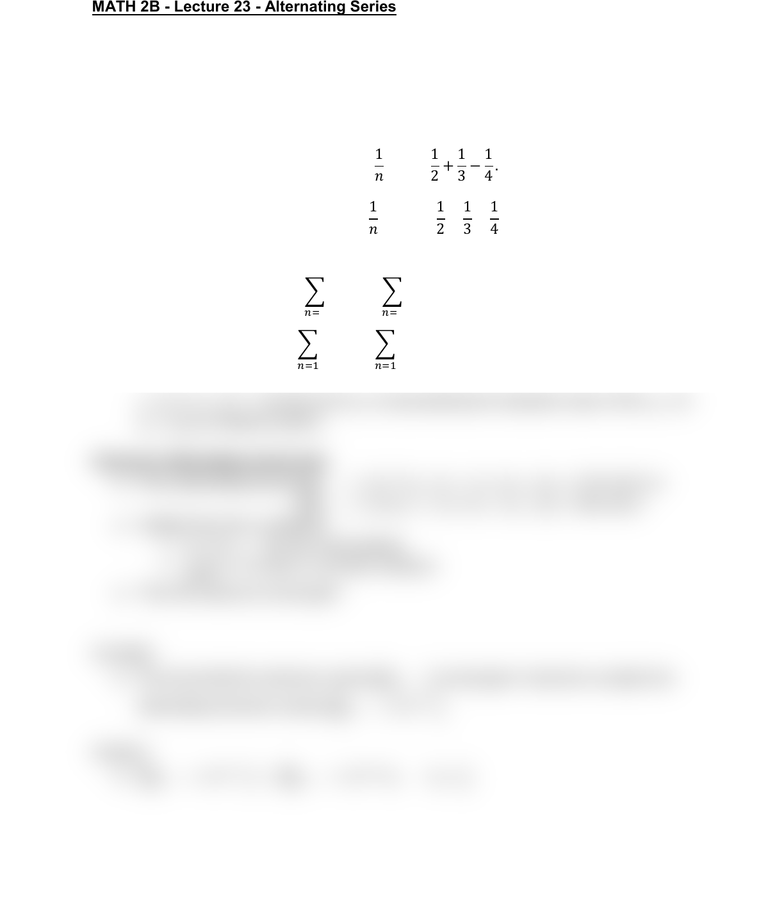Class Notes (1,100,000)
US (460,000)
UC-Irvine (10,000)
MATH (1,000)
MATH 2B (600)
Lecture 23

# MATH 2B Lecture Notes - Lecture 23: Alternating Series Test, Alternating SeriesPremium

Department
Mathematics
Course Code
MATH 2B
Professor
ERJAEE, G.
Lecture
23

This preview shows half of the first page. to view the full 2 pages of the document.02/27/2019
MATH 2B - Lecture 23 - Alternating Series
The convergence tests that we have gone over so far apply only to series with
positive terms. Now we want to learn how to deal with alternating series whose
terms alternate in sign
Example:
 
 

 
 

In general we show alternating series as:


It is very important to understand that the part that causes the sign changes is
or  and the term is representing the absolute value of the . So
is always positive
Theorem: Alternating series test
If the alternating series
 ( for all n) or
for all n)
Satisfy there two conditions:
1.  (for all n decreasing)
2. 
(only 0, not other number)
Then the series is convergent
Example:
We know that the harmonic series
is divergent. Now let’s consider the
alternating harmonic series

Solution:

